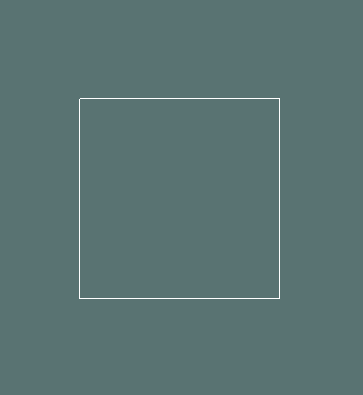Drawing a Cube Outline in GameMaker

Drawing cubes in GameMaker not using the D3D functions. Great for dice and other things.draw_cube_outline() Where each square face line has a width of 1px

_point_h and _point_v should just be a number between 1 and -1 that shows the direction faces should point in.

Usage: draw_cube_outline(100,100,50,0.3,0.3,c_white)

```function draw_cube_outline(_draw_x,_draw_y,_size,_point_h,_point_v,_colour) {

var nodes = [[-1, -1, -1], [-1, -1, 1], [-1, 1, -1], [-1, 1, 1],
[1, -1, -1], [1, -1, 1], [1, 1, -1], [1, 1, 1]];

var edges = [[0, 1], [1, 3], [3, 2], [2, 0], [4, 5], [5, 7], [7, 6],
[6, 4], [0, 4], [1, 5], [2, 6], [3, 7]];

_point_h *= pi
_point_v *= pi

var sinX = sin(_point_h);
var cosX = cos(_point_h);

var sinY = sin(_point_v);
var cosY = cos(_point_v);

var number_of_nodes = array_length(nodes)
for (var i = 0; i < number_of_nodes; ++i) {

var node = nodes[i]

var _x = node;
var _y = node;
var _z = node;

node = _x * cosX - _z * sinX;
node = _z * cosX + _x * sinX;

_z = node;

node = _y * cosY - _z * sinY;
node = _z * cosY + _y * sinY;

nodes[i] = node
};

draw_set_colour(_colour)

var number_of_edges = array_length(edges)
for (var i = 0; i < number_of_edges; ++i) {

var edge = edges[i]

var p1 = nodes[edge];
var p2 = nodes[edge];
draw_line(_draw_x+(p1*_size),_draw_y+(p1*_size),_draw_x+(p2*_size),_draw_y+(p2*_size))

};

}
```

I want to say its not a real 3D model because it has no depth, but when we are always drawing to a 2D screen what even is 3D? Its just done by using sin and cos to find the lengths.draw_cube_width() Where you can define the width of the line of each square face

_point_h and _point_v should just be a number between 1 and -1 that shows the direction faces should point in.

Usage: draw_cube_width(100,100,50,0.3,0.3,c_white,5)

```
function draw_cube_width(_draw_x,_draw_y,_size,_point_h,_point_v,_colour,_width) {

var nodes = [[-1, -1, -1], [-1, -1, 1], [-1, 1, -1], [-1, 1, 1],
[1, -1, -1], [1, -1, 1], [1, 1, -1], [1, 1, 1]];

var edges = [[0, 1], [1, 3], [3, 2], [2, 0], [4, 5], [5, 7], [7, 6],
[6, 4], [0, 4], [1, 5], [2, 6], [3, 7]];

_point_h *= pi
_point_v *= pi

var sinX = sin(_point_h);
var cosX = cos(_point_h);

var sinY = sin(_point_v);
var cosY = cos(_point_v);

var number_of_nodes = array_length(nodes)
for (var i = 0; i < number_of_nodes; ++i) {

var node = nodes[i]

var _x = node;
var _y = node;
var _z = node;

node = _x * cosX - _z * sinX;
node = _z * cosX + _x * sinX;

_z = node;

node = _y * cosY - _z * sinY;
node = _z * cosY + _y * sinY;

nodes[i] = node
};

draw_set_colour(_colour)

var number_of_edges = array_length(edges)
for (var i = 0; i < number_of_edges; ++i) {

var edge = edges[i]

var p1 = nodes[edge];
var p2 = nodes[edge];
draw_line_width(_draw_x+(p1*_size),_draw_y+(p1*_size),_draw_x+(p2*_size),_draw_y+(p2*_size),_width)

draw_circle(_draw_x+(p1*_size),_draw_y+(p1*_size),_width/2,false)
};

}
```

Each corner of the cube has lovely rounded edges.Medium Difficulty
GameMaker
By David Strachan